# NCERT Solutions for Class 10 Maths Chapter 5 Arithmetic Progressions

NCERT Solutions for Class 10 Maths Chapter 5 Arithmetic Progressions

## NCERT Solutions for Class 10 Maths Chapter 5 Arithmetic Progressions Ex 5.1

Ex 5.1 Class 10 Maths Question 1.
In which of the following situations, does the list of numbers involved make an arithmetic progression and why?
(i) The taxi fare after each km when the fare is ₹ 15 for the first km and ₹ 8 for each additional km.
(ii) The amount of air present in a cylinder when a vacuum pump removes 1/4 of the air remaining in the cylinder at a time.
(iii) The cost of digging a well after every meter of digging, when it costs ₹ 150 for the first meter and rises by ₹ 50 for each subsequent meter.
(iv) The amount of money in the account every year, when ₹ 10000 is deposited at compound interest at 8% per annum.
Solution: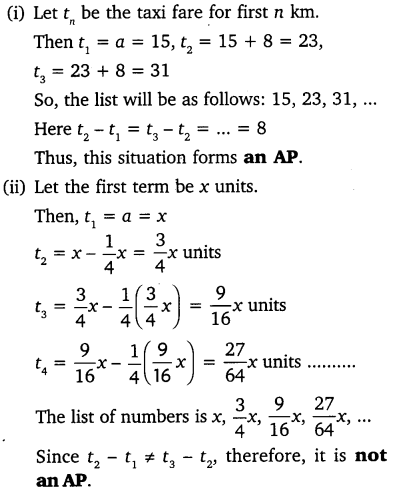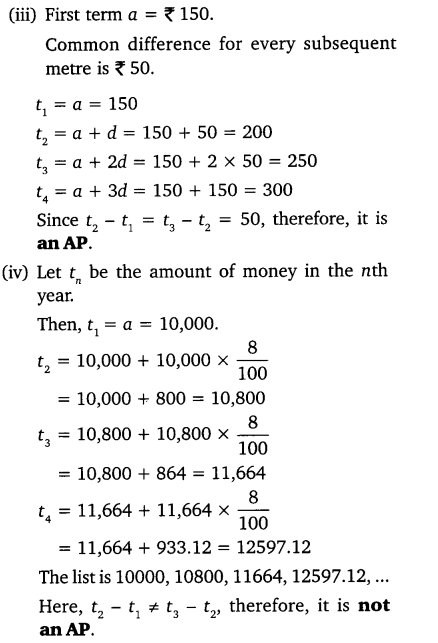Ex 5.1 Class 10 Maths Question 2.
Write first four terms of the AP, when the first term a and the common difference d are given as follows:
(i) a = 10, d = 10
(ii) a = -2, d = 0
(iii) a = 4, d = -3
(iv) a = -1, d = 1/2
(v) a = -1.25, d = -0.25
Solution: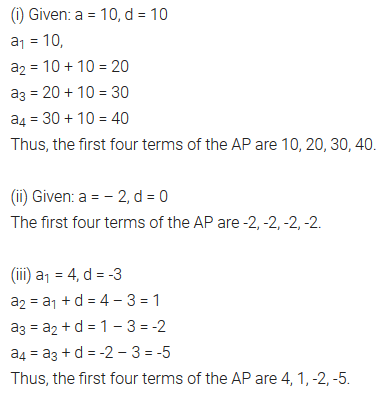You can also download the free PDF of  Ex 5.1 Class 10 Arithmetic Progressions NCERT Solutions or save the solution images and take the print out to keep it handy for your exam preparation.

Ex 5.1 Class 10 Maths Question 3.
For the following APs, write the first term and the common difference:
(i) 3, 1, -1, -3, ……
(ii) -5, -1, 3, 7, ……
(iii) 1/3 , 5/3 , 9/313/3 , ……..
(iv) 0.6, 1.7, 2.8, 3.9, …….
Solution:Ex 5.1 Class 10 Maths Question 4.
Which of the following are APs ? If they form an AP, find the common difference d and write three more terms.
(i) 2, 4, 8, 16, …….
(ii) 2, 5/2 , 3, 7/2 , …….
(iii) -1.2, -3.2, -5.2, -7.2, ……
(iv) -10, -6, -2,2, …..
(v) 3, 3 + √2, 3 + 2√2, 3 + 3√2, …..
(vi) 0.2, 0.22, 0.222, 0.2222, ……
(vii) 0, -4, -8, -12, …..
(viii) 1/2 , 1/2 , 1/2 , 1/2 , …….
(ix) 1, 3, 9, 27, …….
(x) a, 2a, 3a, 4a, …….
(xi) a, a2, a3, a4, …….
(xii) √2, √8, √18, √32, …..
(xiii) √3, √6, √9, √12, …..
(xiv) 12, 32, 52, 72, ……
(xv) 12, 52, 72, 73, ……
Solution:### Class 10 Maths Arithmetic Progressions Mind Maps

#### Arithmetic Progression (AP)

Consider
(i) 1, 2, 3, 4, ……
(ii) 3, 3, 3, 3, …..
(i) and (ii) are the sequence of numbers, each number in these sequences is called a term.

An arithmetic progression (AP) is a sequence of numbers in which each term is obtained by adding a fixed number ‘d’ to the preceeding term, except the first term.
The fixed number is called the common difference. It can be positive, negative or zero.
Any Arithmetic progression can be represented as :
a, a + d, a + 2d, a + 3d,…..
where ‘a’ is the first term and ‘d’ is the common difference. Arithmetic progressions which does not have a last term are called Infinite Arithmetic Progression. e.g.:
6, 9, 12, 15,…….

#### Formula for common Difference (d)

A sequence of numbers a1, a2, a3…. is an AP if the difference a2 – a1, a3 – a2, a4 – a3…. gives the same value, i.e. if ak+1 – ak is the same for different values of k. The difference (ak+1 – ak) is called common difference (d). Here ak+1 and ak are the (k + 1)th and kth terms respectively.
∴ d = a2 – a1 = a3 – a2 = a4 – a3

#### nth Term (or General Term) of an Arithmetic Progressions

In an AP, with first term ‘a’ and common difference d, the nth term(or the general term) is given by,
an = a + (n – 1)d
Note that an AP can be finite or infinite according to as the number of terms are finite or infinite.
If there are m terms in an AP then am is the last term and is sometimes denoted by ‘l’.

#### Sum of the FIRST ‘n’ Terms of an A.P.

(i) The sum of the first n terms of an A.P. is given by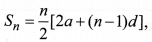where a is the first term and d is the common difference
(ii) If l is the last term of the finite A.P. say the nth term, then the sum of all terms of the A.P. is given by,Note that sum of first n positive integers is given by#### Arithmetic Mean Between Two Numbers

If a, b, c are in AP. Then b is called the arithmetic mean of a and c and is given by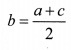## NCERT Solutions for Class 10 Maths Chapter 5 Arithmetic Progressions Ex 5.2

NCERT Solutions for Class 10 Maths Chapter 5 Arithmetic Progressions Ex 5.2 are part of NCERT Solutions for Class 10 Maths. Here we have given NCERT Solutions for Class 10 Maths Chapter 5 Arithmetic Progressions Exercise 5.2

Ex 5.2 Class 10 Maths Question 1.
Fill in the blanks in the following table, given that a is the first term, d the common difference and the nth term of the AP:Solution: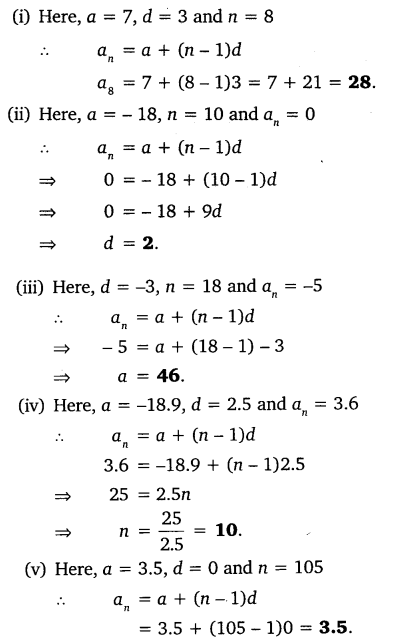Ex 5.2 Class 10 Maths Question 2.
Choose the correct choice in the following and justify:
(i) 30th term of the AP: 10, 7, 4, …, is
(a) 97
(b) 77
(c) -77
(d) -87

(ii) 11th term of the AP: -3, 1/2 , 2, …, is
(a) 28
(b) 22
(c) -38
(d) -48
Solution:Ex 5.2 Class 10 Maths Question 3.
In the following APs, find the missing terms in the boxes: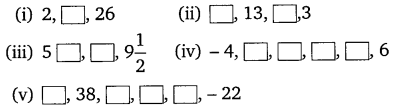Solution: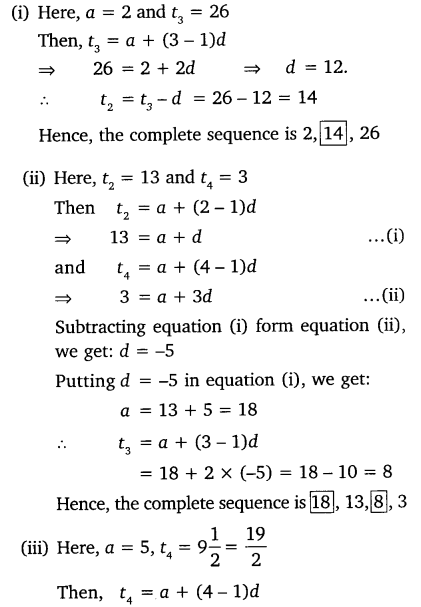Ex 5.2 Class 10 Maths Question 4.
Which term of the AP: 3, 8, 13, 18, …, is 78?
Solution: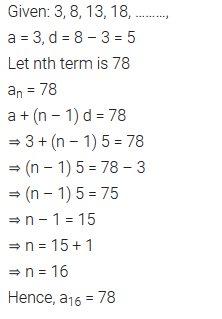Ex 5.2 Class 10 Maths Question 5.
Find the number of terms in each of the following APs:
(i) 7, 13, 19, …, 205
(ii) 18, 15 1/2, 13, …, -47
Solution: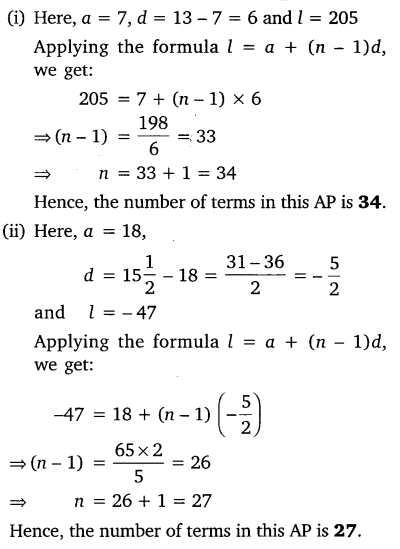Ex 5.2 Class 10 Maths Question 6.
Check, whether -150 is a term of the AP: 11, 8, 5, 2, ….
Solution: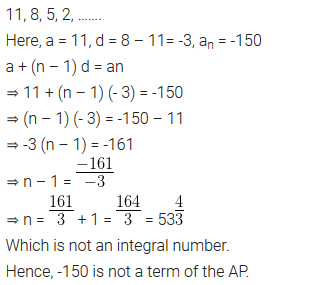Ex 5.2 Class 10 Maths Question 7.
Find the 31st term of an AP whose 11th term is 38 and the 16th term is 73.
Solution:Ex 5.2 Class 10 Maths Question 8.
An AP consists of 50 terms of which 3rd term is 12 and the last term is 106. Find the 29th term.
Solution:Ex 5.2 Class 10 Maths Question 9.
If the 3rd and the 9th term of an AP are 4 and -8 respectively, which term of this AP is zero?
Solution: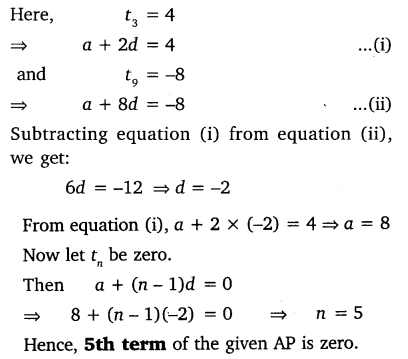Ex 5.2 Class 10 Maths Question 10.
The 17th term of an AP exceeds its 10th term by 7. Find the common difference.
Solution:Ex 5.2 Class 10 Maths Question 11.
Which term of the AP: 3, 15, 27, 39, … will be 132 more than its 54th term?
Solution: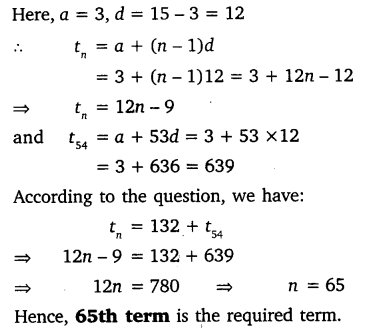Ex 5.2 Class 10 Maths Question 12.
Two APs have the same common difference. The difference between their 100th terms is 100, what is the difference between their 1000th terms?
Solution:Ex 5.2 Class 10 Maths Question 13.
How many three-digit numbers are divisible by 7?
Solution:Ex 5.2 Class 10 Maths Question 14.
How many multiples of 4 lie between 10 and 250?
Solution:Ex 5.2 Class 10 Maths Question 15.
For what value of n, the nth term of two APs: 63, 65, 61,… and 3, 10, 17,… are equal?
Solution: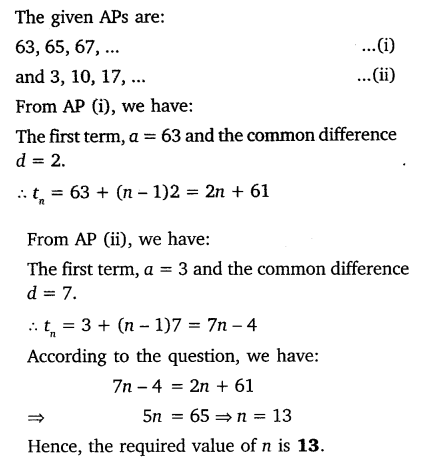Ex 5.2 Class 10 Maths Question 16.
Determine the AP whose 3rd term is 16 and 7th term exceeds the 5th term by 12.
Solution:Ex 5.2 Class 10 Maths Question 17.
Find the 20th term from the last term of the AP: 3, 8, 13, …, 253.
Solution:Ex 5.2 Class 10 Maths Question 18.
The sum of the 4th and 8th terms of an AP is 24 and the sum of the 6th and 10th terms is 44. Find the first three terms of the AP.
Solution: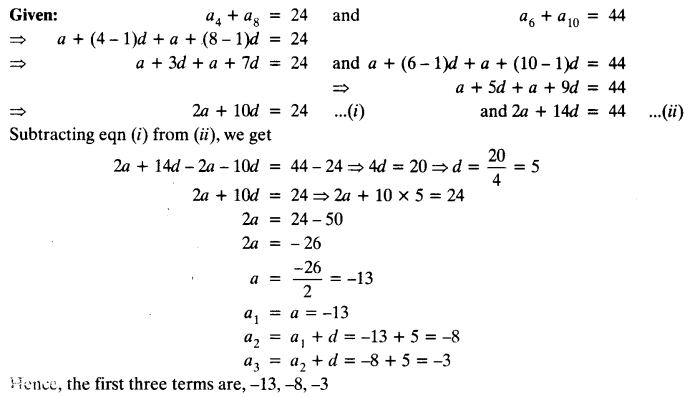Ex 5.2 Class 10 Maths Question 19.
Subba Rao started work in 1995 at an annual salary of ₹ 5000 and received an increment of ₹ 200 each year. In which year did his income reach ₹ 7000 ?
Solution: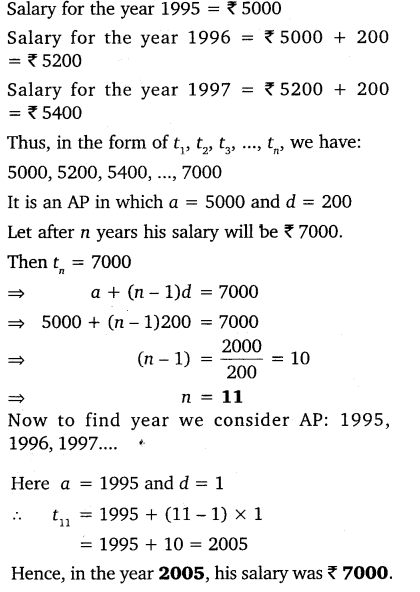Ex 5.2 Class 10 Maths Question 20.
Ramkali saved ₹ 5 in the first week of a year and then increased her weekly saving by ₹ 1.75. If in the nth week, her weekly saving become ₹ 20.75, find n.
Solution: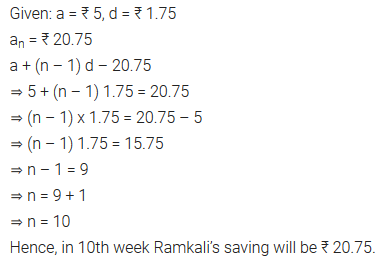## NCERT Solutions for Class 10 Maths Chapter 5 Arithmetic Progressions Ex 5.3

Ex 5.3 Class 10 Maths Question 1.
Find the sum of the following APs:
(i) 2, 7, 12,…… to 10 terms.
(ii) -37, -33, -29, …… to 12 terms.
(iii) 0.6, 1.7, 2.8, ……, to 100 terms.
(iv) 1/151/121/10, …….., to 11 terms.
Solution:Ex 5.3 Class 10 Maths Question 2.
Find the sums given below:
(i) 7 + 10 1/2 + 14 + … + 84
(ii) 34 + 32 + 30 + … + 10
(iii) -5 + (-8) + (-11) + ….. + (-230)
Solution:Ex 5.3 Class 10 Maths Question 3.
In an AP:
(i) given a = 5, d = 3, an = 50, find n and Sn.
(ii) given a = 7, a13 = 35, find d and S13.
(iii) given a12 = 37, d = 3, find a and S12.
(iv) given a3 = -15, S10 = 125, find d and a10.
(v) given d = 5, S9 = 75, find a and a9.
(vi) given a = 2, d = 8, Sn = 90, find n and an.
(vii) given a = 8, an = 62, Sn = 210, find n and d.
(viii) given an = 4, d = 2, Sn = -14, find n and a.
(ix) given a = 3, n = 8, S = 192, find d.
(x) given l = 28, S = 144, and there are total 9 terms. Find a.
Solution: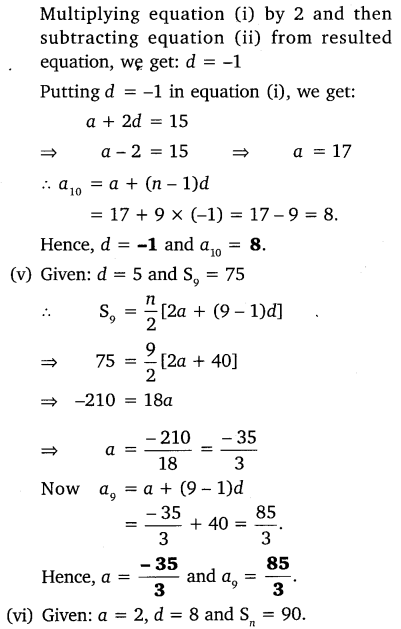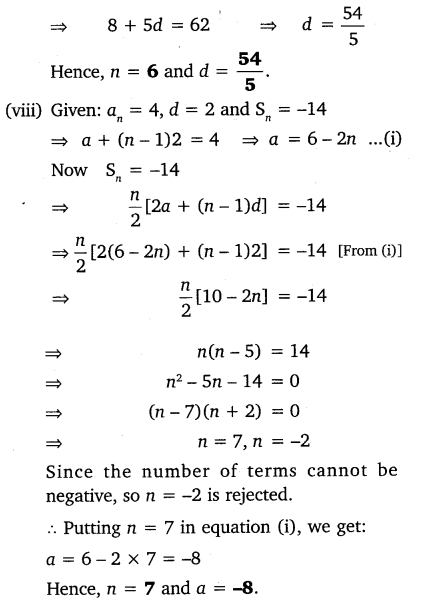Ex 5.3 Class 10 Maths Question 4.
How many terms of AP: 9, 17, 25, … must be taken to give a sum of 636?
Solution: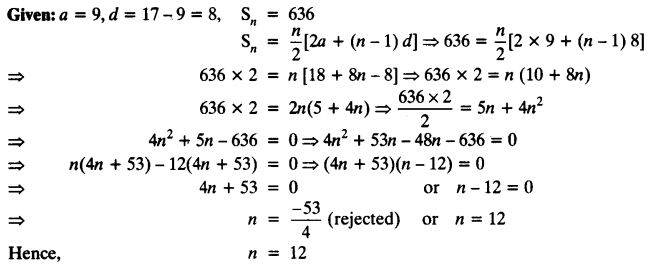Ex 5.3 Class 10 Maths Question 5.
The first term of an AP is 5, the last term is 45 and the sum is 400. Find the number of terms and the common difference.
Solution:Ex 5.3 Class 10 Maths Question 6.
The first and the last terms of an AP are 17 and 350 respectively. If the common difference is 9, how many terms are there and what is their sum?
Solution: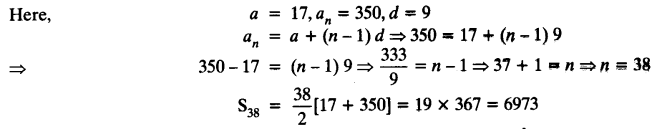Ex 5.3 Class 10 Maths Question 7.
Find the sum of first 22 terms of an AP in which d = 7 and 22nd term is 149.
Solution:Ex 5.3 Class 10 Maths Question 8.
Find the sum of first 51 terms of an AP whose second and third terms are 14 and 18 respectively.
Solution: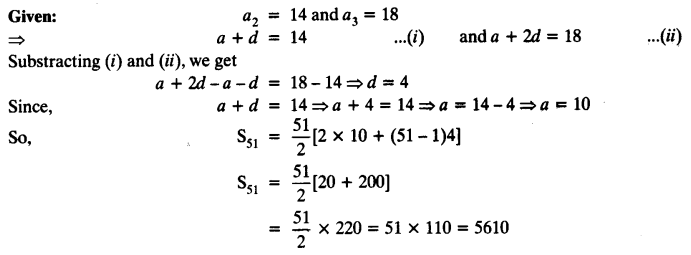Ex 5.3 Class 10 Maths Question 9.
If the sum of first 7 terms of an AP is 49 and that of 17 terms is 289, find the sum of first n terms.
Solution: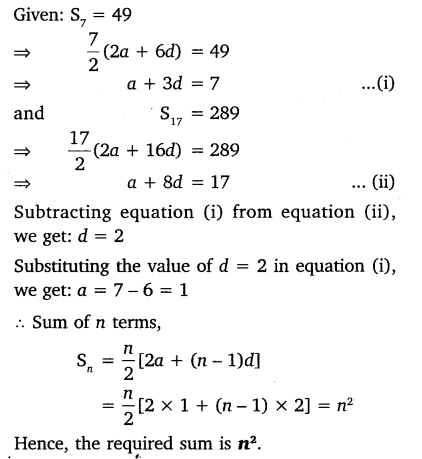Ex 5.3 Class 10 Maths Question 10.
Show that a1, a2, ……. an,…… form an AP where an is defined as below:
(i) an = 3 + 4n
(ii) an = 9 – 5n
Also find the sum of the first 15 terms in each case.
Solution:Ex 5.3 Class 10 Maths Question 11.
If the sum of the first n terms of an AP is 4n – n2, what is the first term (that is S1)? What is the sum of first two terms? What is the second term? Similarly, find the 3rd, the 10th and the nth terms.
Solution:Ex 5.3 Class 10 Maths Question 12.
Find the sum of the first 40 positive integers divisible by 6.
Solution:Ex 5.3 Class 10 Maths Question 13.
Find the sum of the first 15 multiples of 8.
Solution:Ex 5.3 Class 10 Maths Question 14.
Find the sum of the odd numbers between 0 and 50.
Solution:Ex 5.3 Class 10 Maths Question 15.
A contract on construction job specifies a penalty for delay of completion beyond a certain date as follows:
₹ 200 for the first day, ₹ 250 for the second day, ₹ 300 for the third day, etc. the penalty for each succeeding day being ₹ 50 more than for the preceding day. How much money the contractor has to pay as penalty, if he has delayed the work by 30 days?
Solution:Ex 5.3 Class 10 Maths Question 16.
A sum of ₹ 700 is to be used to give seven cash prizes to students of a school for their overall academic performance. If each prize is ₹ 20 less than its preceding prize, find the value of each of the prizes.
Solution:Ex 5.3 Class 10 Maths Question 17.
In a school, students thought of planting trees in and around the school to reduce air pollution. It was decided that the number of trees, that each section of each class will plant, will be the same as the class, in which they are studying, eg. a section of Class I will plant 1 tree, a section of Class II will plant 2 trees and so on till Class XII. There are three sections of each class. How many trees will be planted by the students?
Solution:Ex 5.3 Class 10 Maths Question 18.
A spiral is made up of successive semicircles, with centres alternately at A and B, starting with centre at A, of radii 0.5 cm, 1.0 cm, 1.5 cm, 2.0 cm,… as shown in figure. What is the total length of such a spiral made up of thirteen consecutive semicircles?
(Take π = 22/7)
[Hint: Length of successive semicircles is l1, l2, l3, l4, … with centres at A, B, respectively.]Solution: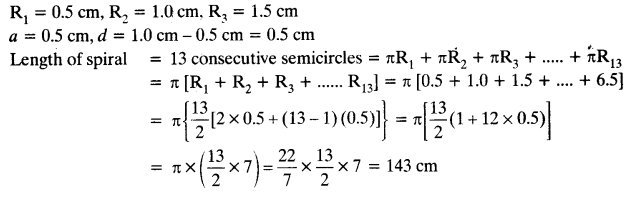Ex 5.3 Class 10 Maths Question 19.
200 logs are stacked in the following manner 20 logs in the bottom row, 19 in the next row, 18 in the row next to it and so on (see Figure). In how many rows are the 200 logs placed and how many logs are in the top row?Solution:Ex 5.3 Class 10 Maths Question 20.
In a potato race, a bucket is placed at the starting point, which is 5 m from the first potato, and the other potatoes are placed 3 m apart in a straight line. There are ten potatoes in the line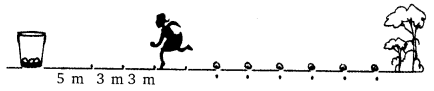A competitor starts from the bucket, picks up the nearest potato, runs back with it, drops it in the bucket, runs back to pick up the next potato, runs to the bucket to drop it in, and she continues in the same way until all the potatoes are in the bucket. What is the total distance the competitor has to run?
Solution:## NCERT Solutions for Class 10 Maths Chapter 5 Arithmetic Progressions Ex 5.4

Ex 5.4 Class 10 Maths Question 1.
Which term of the AP: 121, 117. 113, ….., is its first negative term?
Solution:Ex 5.4 Class 10 Maths Question 2.
The sum of the third term and the seventh term of an AP is 6 and their product is 8. Find the sum of first sixteen terms of the AP?
Solution: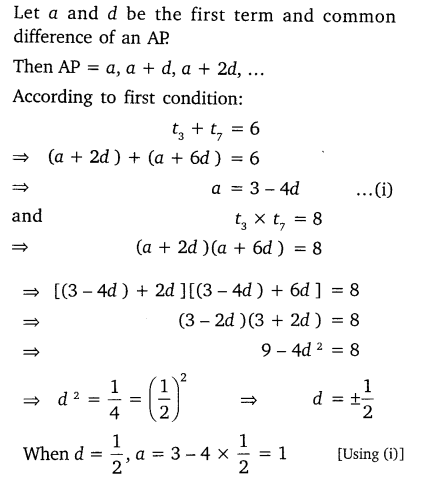Ex 5.4 Class 10 Maths Question 3.
A ladder has rungs 25 cm apart. The rungs decrease uniformly in length from 45 cm at the bottom to 25 at the top. If the top and the bottom rungs are 2 1/2m apart, what is the length of the wood required for the rungs?Solution:Ex 5.4 Class 10 Maths Question 4.
The houses of a row are numbered consecutively from 1 to 49. Show that there is value of x such that the sum of the numbers of the houses preceding the house numbered x is equal to the sum of the numbers of the houses following it. Find this value of x.
Solution:Ex 5.4 Class 10 Maths Question 5.
A small terrace at a football ground comprises of 15 steps each of which is 50  m long and built of solid concrete. Each step has a rise of 1/2m and a tread of 1/2m. Calculate the total volume of concrete required to build the terrace.Solution:## Arithmetic Progressions Class 10 Extra Questions Maths Chapter 5

Extra Questions for Class 10 Maths Chapter 5 Arithmetic Progressions. According to new CBSE Exam Pattern, MCQ Questions for Class 10 Maths Carries 20 Marks.## CBSE Class 10 Maths Notes Chapter 5 Arithmetic Progressions

SEQUENCE:
A sequence is an arrangement of numbers in a definite order and according to some rule.
Example: 1, 3, 5,7,9, … is a sequence where each successive item is 2 greater than the preceding term and 1, 4, 9, 16, 25, … is a sequence where each term is the square of successive natural numbers.

TERMS :
The various numbers occurring in a sequence are called ‘terms’. Since the order of a sequence is fixed, therefore the terms are known by the position they occupy in the sequence.
Example: If the sequence is defined asARITHMETIC PROGRESSION (A.P.):
An Arithmetic progression is a special case of a sequence, where the difference between a term and its preceding term is always constant, known as common difference, i.e., d. The arithmetic progression is abbreviated as A.P.

The general form of an A.P. is
∴ a, a + d, a + 2d,… For example, 1, 9, 11, 13.., Here the common difference is 2. Hence it is an A.P.

In an A.P. with first term a and common difference d, the nth term (or the general term) is given
by .
an = a + (n – 1)d.
…where [a = first term, d = common difference, n = term number
Example: To find seventh term put n = 7
∴ a7 = a + (7 – 1)d or a7 = a + 6d

The sum of the first n terms of an A.P. is given by
Sn = n/2[2a + (n – 1)d] or n/2[a + 1]
where, 1 is the last term of the finite AP.

If a, b, c are in A.P. then b = a+c/2 and b is called the arithmetic mean of a and c.

## Important Questions for Class 10 Maths Chapter 5 Arithmetic Progressions

### Arithmetic Progressions Class 10 Important Questions Very Short Answer (1 Mark)

Question 1.
Find the common difference of the AP 1/p,1p/p,12p/p,. (2013D)
Solution:
The common difference,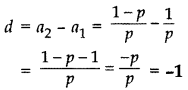Question 2.
Find the common difference of the A.P. 1/2b,16b/2b,112b/2b,. (2013D)
Solution:
The common difference, d = a2 – a1 = 16b/2b1/2b
16b1/2b=6b/2b = -3

Question 3.
Find the common difference of the A.P. 1/3q,16q/3q,112q/3q,. (2013D)
Solution:
Common difference, d = a2 – a1 = 16q/3q1/3q
16q1/3q=6q/3q = -2

Question 4.
Calculate the common difference of the A.P. 1/b,3b/3b,32b/3b,. (2013D)
Solution:
Common difference, d = a2 – a13b/3b1/b
3b3/3b=b/3b=1/3

Question 5.
Calculate the common difference of the A.P. 1/3,13b/3,16b/3, (2013OD)
Solution:
Common difference, d = a2 – a1 = 13b/31/3

13b1/3=3b/3 = -b

Question 6.
A thief runs with a uniform speed of 100 m/minute. After one minute a policeman runs after the thief to catch him. He goes with a speed of 100 m/minute in the first minute and increases his speed by 10 m/minute every succeeding minute. After how many minutes the policeman will catch the thief. (2016D)
Solution:
Let total time ben minutes. Total distance covered by thief in n minutes
= Speed × Time
= 100 × n = 100 n metres
Total distance covered by policeman⇒ (n – 1) (200 + 10n – 20) = 200n
⇒ (n – 1) [10n + 180) = 200n
⇒ 10n2 + 180n – 10n – 180 – 200n = 0
⇒ 10n2 – 30n – 180 = 0
⇒ n2 – 3n – 18 = 0 … [Dividing both sides by 10
⇒ n2 – 6n + 3n – 18 = 0
⇒ n(n – 6) + 3(n – 6) = 0
⇒ (n + 3) (n – 6) = 0
⇒ n + 3 = 0 or n – 6 = 0
⇒ n = -3 or n = 6
But n (time) can not be negative.
∴ Time taken by policeman to catch the thief
= n – 1 = 6 – 1 = 5 minutes

Question 7.
A thief, after committing a theft, runs at a uniform speed of 50 m/minute. After 2 minutes, a policeman runs to catch him. He goes 60 m in first minute and increases his speed by 5 m/ minute every succeeding minute. After how many minutes, the policeman will catch the thief? (2016D)
Solution:
Let total time be n minutes.
Total distance covered by thief in n minutes
= Speed × Time
= (50 × n) metres = 50 n metres
Total distance covered by policeman⇒ (n – 2) (120 + 5n – 15) = 100n
⇒ (n – 2) [5n + 105) = 100n
⇒ 5n2 + 105n – 10n – 210 – 100n = 0
⇒ 5n2 – 5n – 210 = 0
⇒ n2 – n – 42 = 0 …[Dividing both sides by 5
⇒ n2 – 7n + 6n – 42 = 0
⇒ n(n – 7) + 6(n – 7) = 0
⇒ (n – 7) (n + 6) = 0
⇒ n – 7 = 0 or n + 6 = 0
⇒ n = 7 or n = -6 (reject)
But n (time) can not be negative.
∴ Time taken by policeman to catch the thief
= n – 2 = 7 – 2 = 5 minutes

Question 8.
The houses in a row are numbered conse cutively from 1 to 49. Show that there exists a value of X such that sum of numbers of houses preceding the house numbered X is equal to sum of the numbers of houses following X. (2016OD)
Solution:
Here the A.P. is 1, 2, 3, …., 49
Here a = 1, d = 1, an = 49Now,
⇒ (X – 1)2 (2 + (X – 2)) = 49(2 + 48) – X[2 + (x – 1)]
⇒ (X – 1). X = 2,450 – X(X + 1)
⇒ x2 – X = 2,450 – X2 – X
⇒ X2 – X + X2 + X = 2,450
⇒ 2X2 = 2,450
⇒ X2 = 1,225
∴ X = +√1,225 = 35 …[X can not be -ve

## SabDekho

The Complete Educational Website